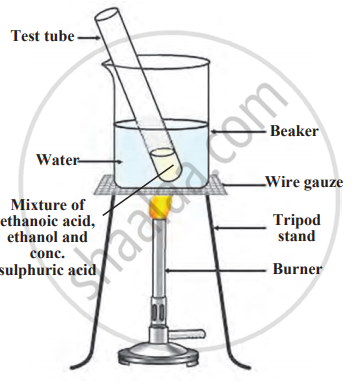# Observe the figure and write the answers to the following questions. 1. Write the name of the reaction shown in the following figure. 2. Write the above chemical reaction in the - Science and Technology 1

Observe the figure and write the answers to the following questions.1. Write the name of the reaction shown in the following figure.
2. Write the above chemical reaction in the form of a balanced equation.
3. Write the name of the product produced in the above reaction, write a use.
4. Write the name of the catalyst used in the above reaction.

#### Solution

1. The reaction shown in the figure is esterification.
2. Balanced equation of the reaction between ethanol and ethanoic acid is as follows:
$\ce{\underset{\text{Ethanol}}{C2H5OH} + \underset{\text{Ethanoic acid}}{CH3COOH} ->[Conc.][H2SO4] \underset{\text{Ethyl ethanoate}}{CH3COOC2H5} + \underset{\text{Water}}{H2O}}$
3. The product formed is ethyl ethanoate.
Use: It is used for making fragrances and flavouring agents.
4. The catalyst used in the reaction is concentrated sulphuric acid.
Concept: Ethanoic Acid
Is there an error in this question or solution?

Share